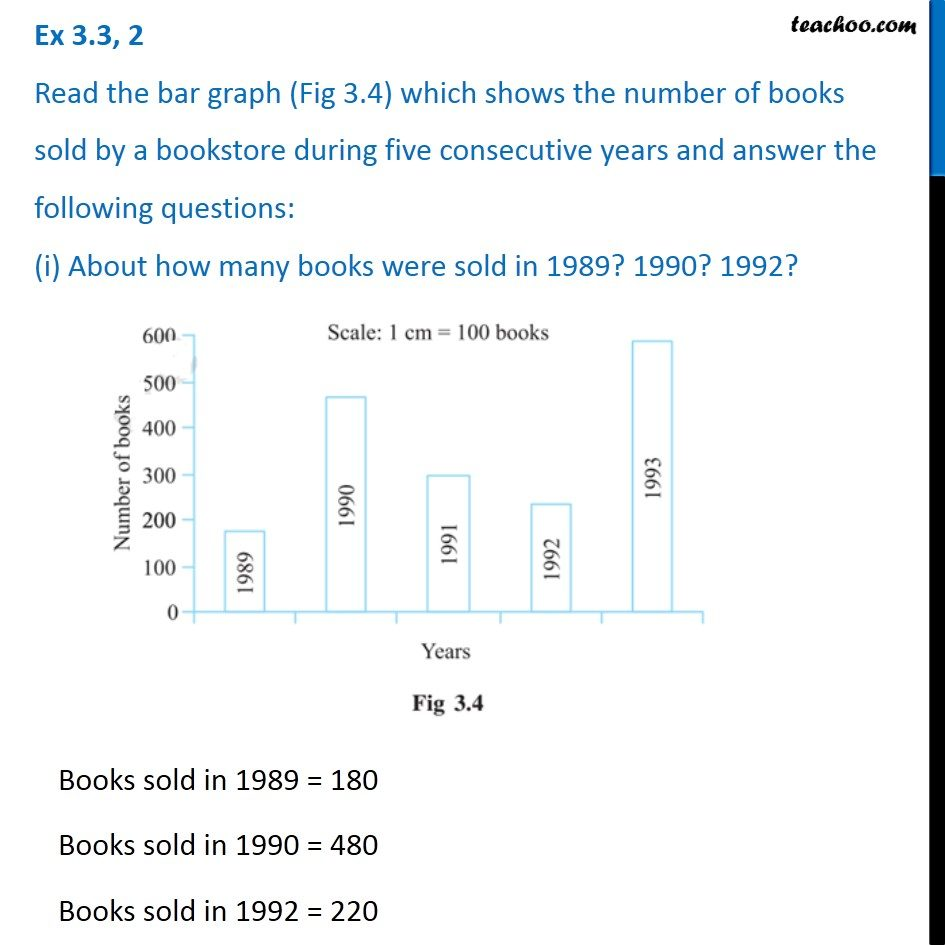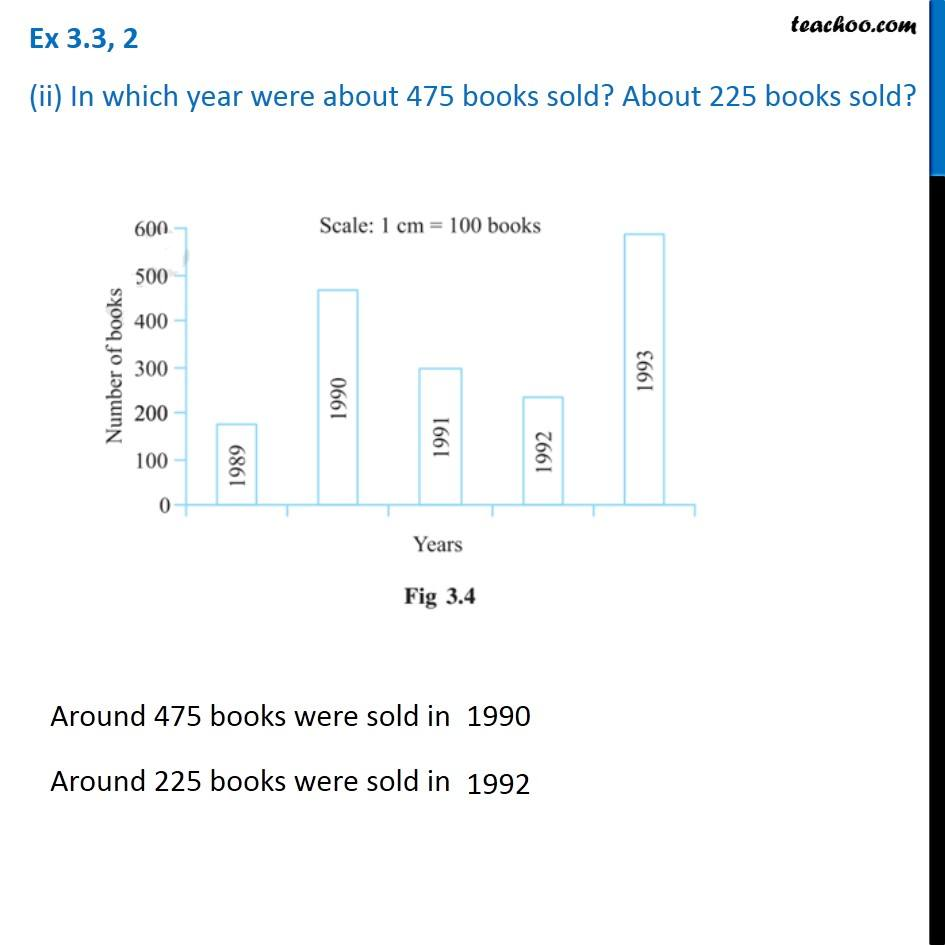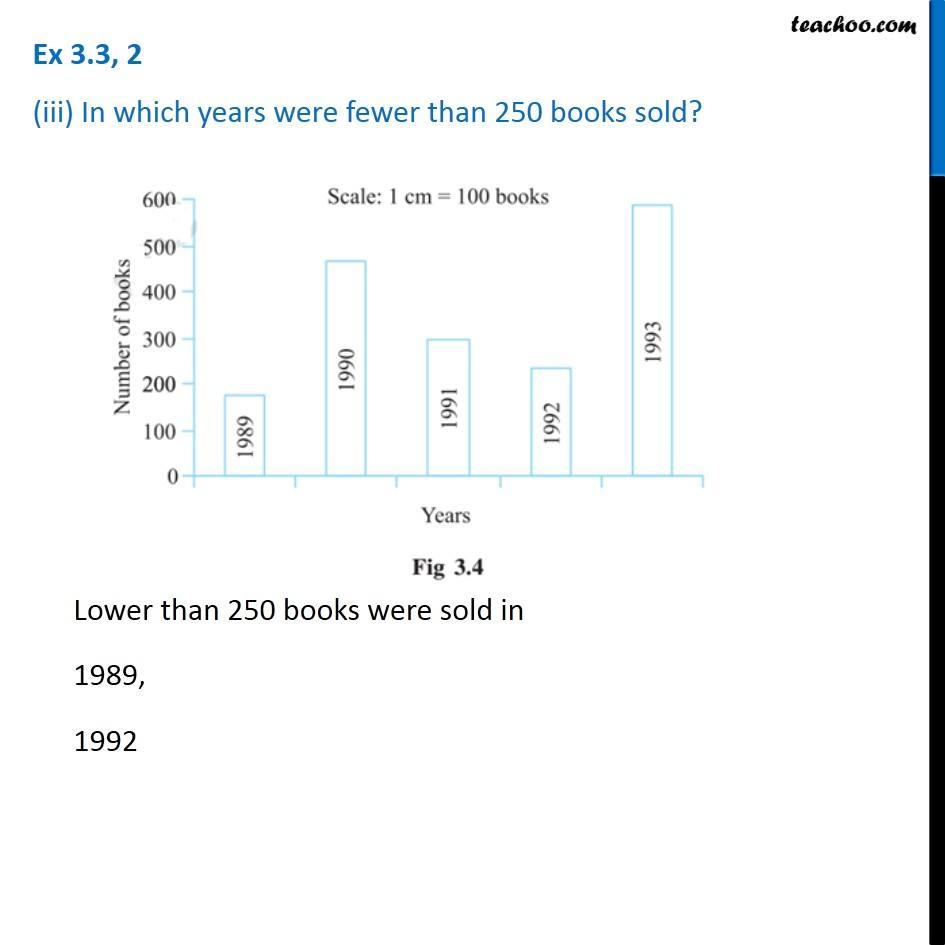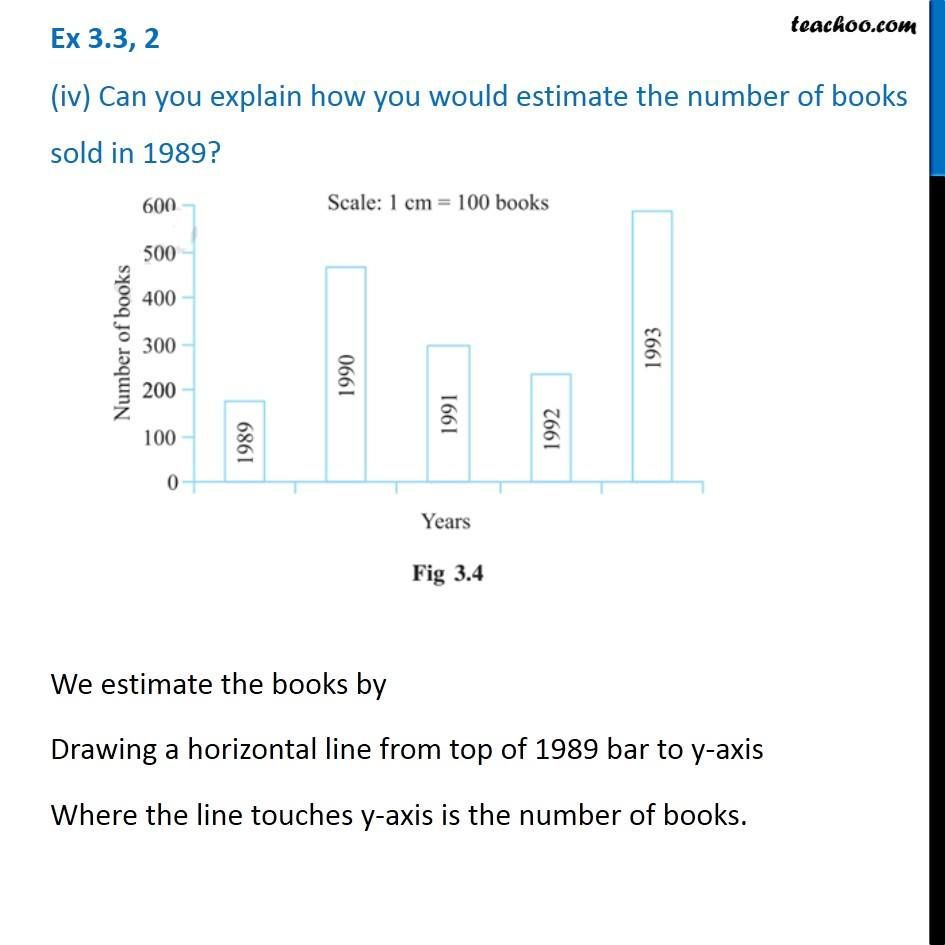1. Chapter 3 Class 7 Data Handling
2. Serial order wise
3. Ex 3.3

Transcript

Ex 3.3, 2 Read the bar graph (Fig 3.4) which shows the number of books sold by a bookstore during five consecutive years and answer the following questions: (i) About how many books were sold in 1989? 1990? 1992?Books sold in 1989 = 180 Books sold in 1990 = 480 Books sold in 1992 = 220 Ex 3.3, 2 (ii) In which year were about 475 books sold? About 225 books sold?Around 475 books were sold in Around 225 books were sold in Ex 3.3, 2 (iii) In which years were fewer than 250 books sold? Lower than 250 books were sold in 1989, 1992 We estimate the books by Drawing a horizontal line from top of 1989 bar to y-axis Where the line touches y-axis is the number of books.

Ex 3.3